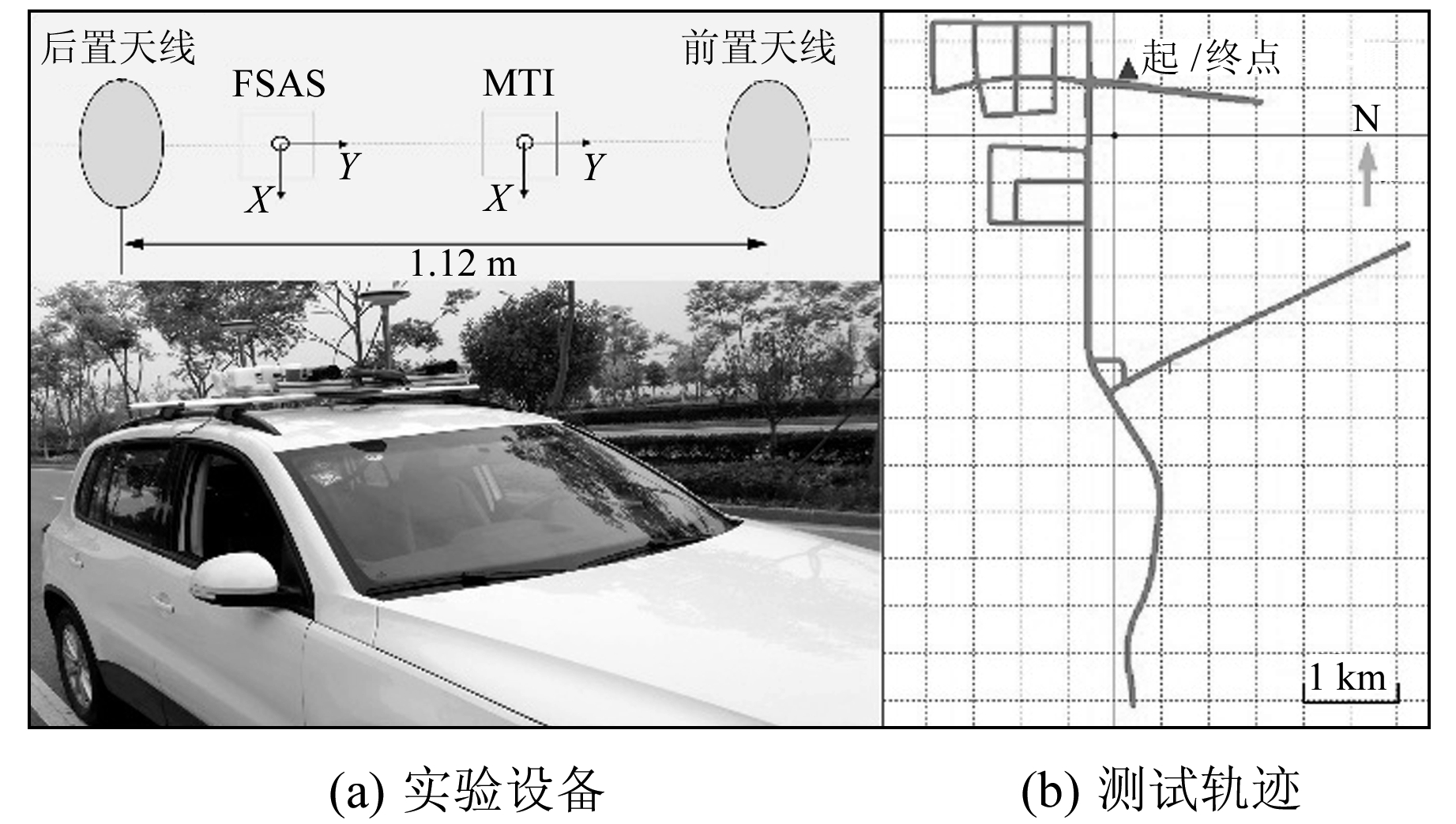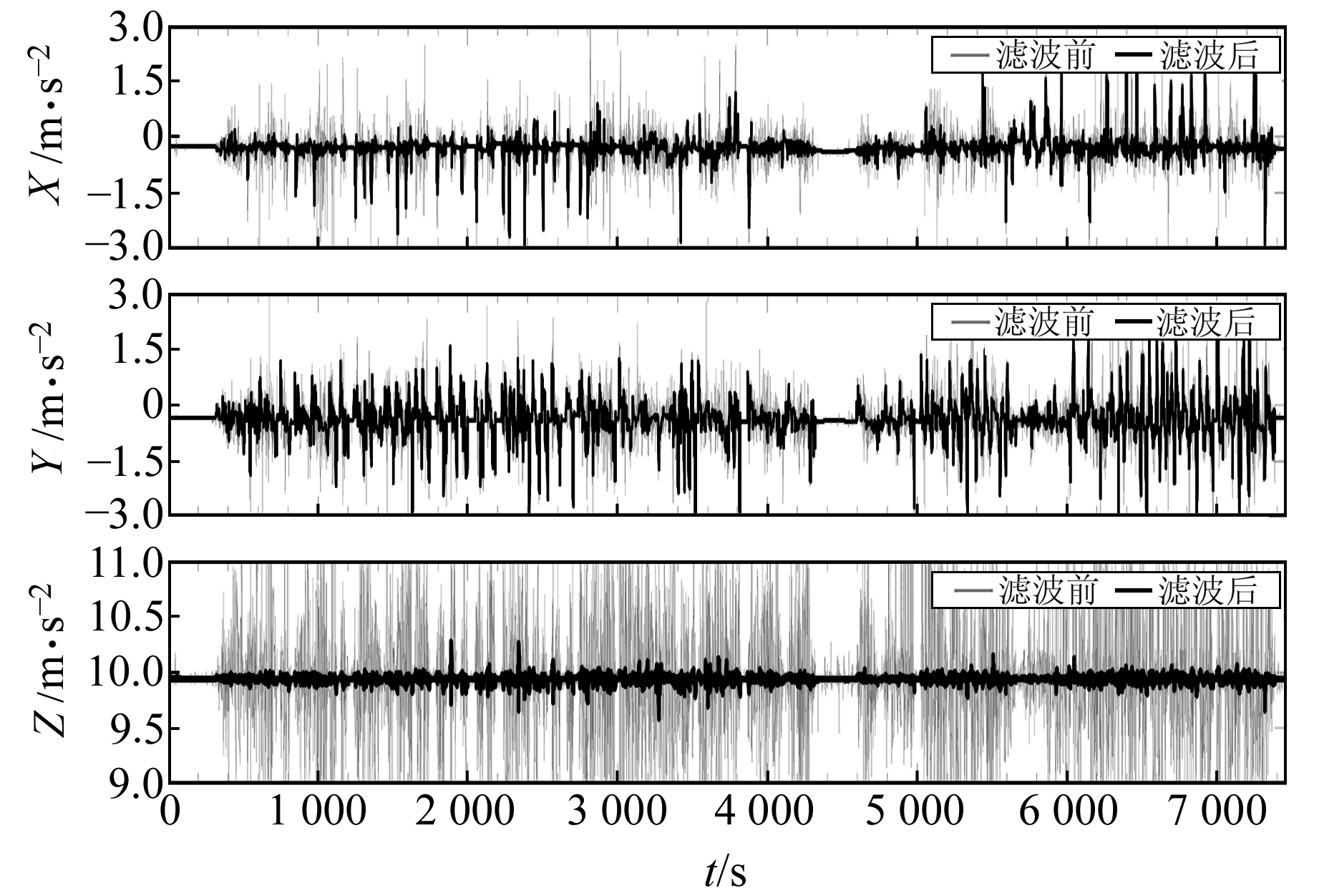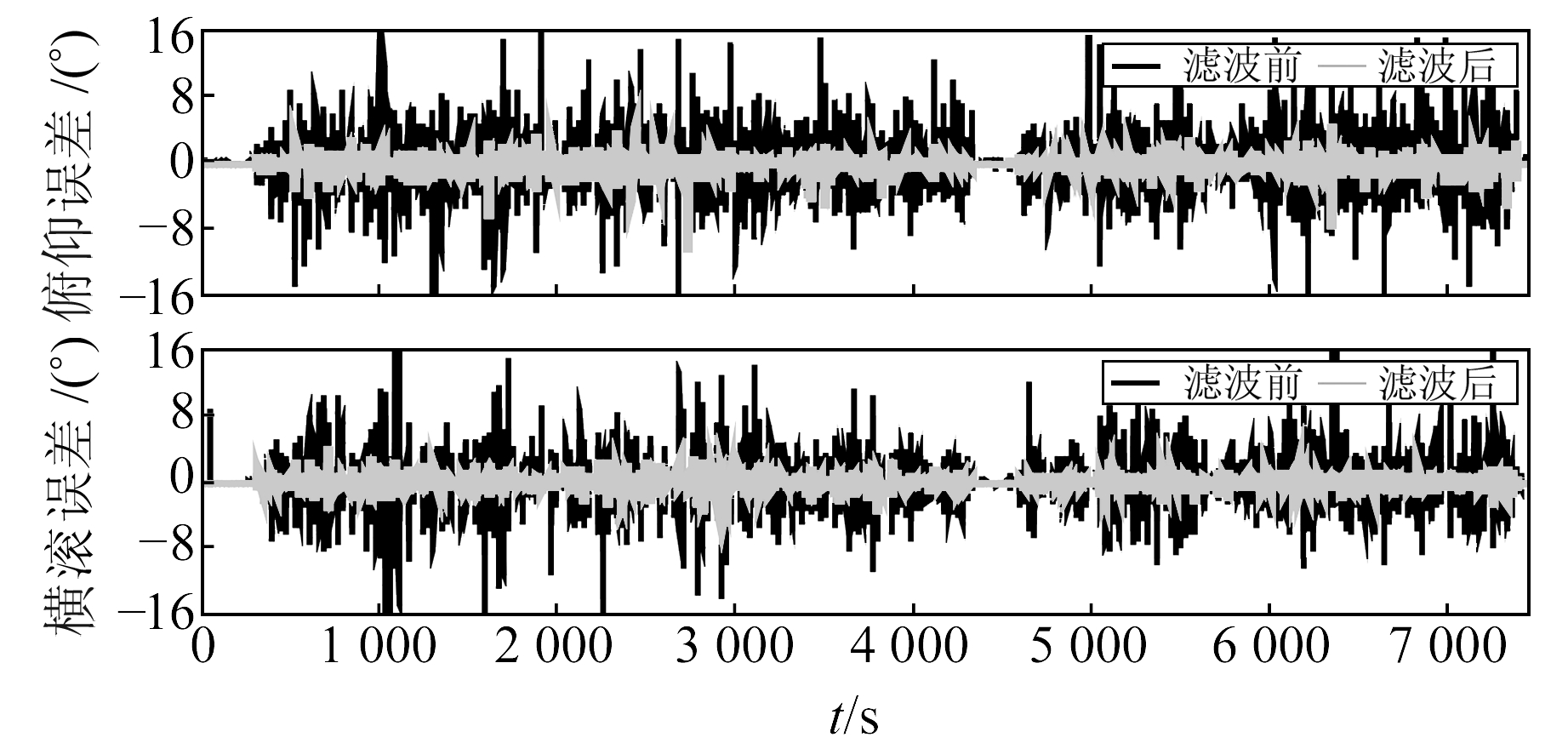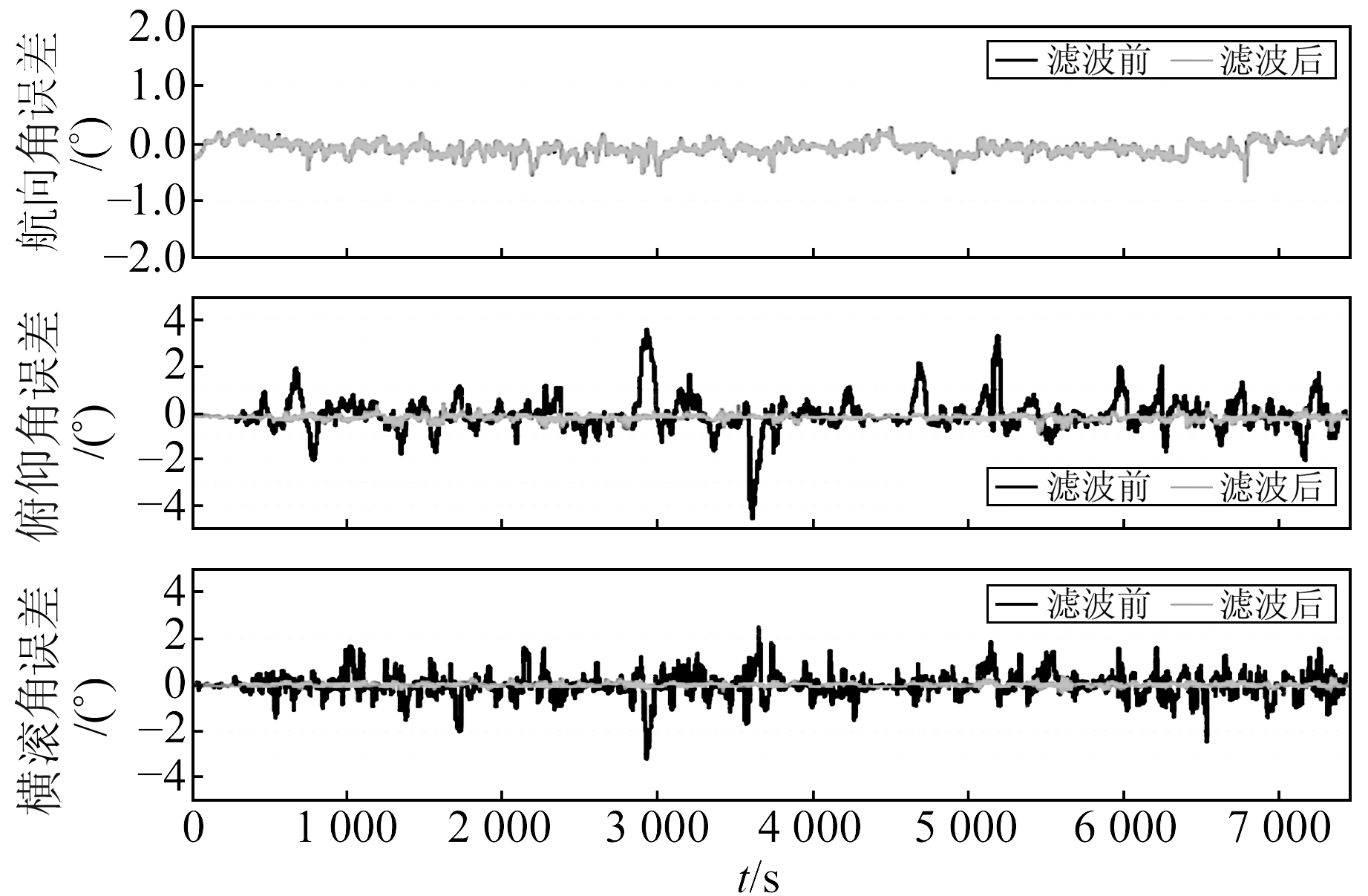﻿ 利用Butterworth滤波器平滑加速度计的双天线GNSS/MEMS组合测姿文章快速检索 高级检索
 大地测量与地球动力学2020, Vol. 40Issue (1): 51-55  DOI: 10.14075/j.jgg.2020.01.010### 引用本文HU Zengke, ZHU Feng, LIU Wanke. Dual Antenna GNSS/MEMS Integration Attitude Determination with Butterworth Filter Smoothed Accelerometer[J]. Journal of Geodesy and Geodynamics, 2020, 40(1): 51-55.### Foundation support

National Key Research and Development Program of China, No. 2016YFB0501803.

### 第一作者简介

HU Zengke, postgraduate, majors in GNSS/MEMS integration navigation and attitude determination, E-mail: zkhusgg@whu.edu.cn.

### 文章历史

1. 武汉大学测绘学院，武汉市珞喻路129号，430079;
2. 武汉大学地球空间环境与大地测量教育部重点实验室，武汉市珞喻路129号，430079

1 组合测姿原理 1.1 双天线GNSS/MEMS组合测姿

 $\left\{\begin{array}{l} {\boldsymbol{{\dot \phi }}=-\boldsymbol{R}_{b}^{e}\left(\boldsymbol{b}_{g}+\boldsymbol{S}_{g} \boldsymbol{\omega}_{b}^{b}\right)+\boldsymbol{\phi} \boldsymbol{\omega}_{i k}^{e}+\boldsymbol{\xi}_{j}} \\ {\dot{\boldsymbol{b}}_{g}=-1 / \tau_{g} \cdot \boldsymbol{b}_{g}+\boldsymbol{\xi}_{g}} \\ {\dot{\boldsymbol{S}}_{g}=-1 / \tau_{s_{c}} \cdot \boldsymbol{S}_{g}+\boldsymbol{\xi}_{s_{g}}} \end{array}\right.$ (1)

 $\boldsymbol{R}_{b}^{l}=\boldsymbol{R}_{e}^{l} \boldsymbol{R}_{b}^{e}=\boldsymbol{R}_{e}^{l}(\boldsymbol{I}+\boldsymbol{\phi} \times) \widetilde{\boldsymbol{R}}_{b}^{e}$ (2)

 $\delta \mathit{\boldsymbol{z}} = \left[ {\begin{array}{*{20}{l}} {\delta \alpha }\\ {\delta \gamma }\\ {\delta \beta } \end{array}} \right] = \left[ {\begin{array}{*{20}{l}} {\alpha - {\alpha _1}}\\ {\gamma - {\gamma _1}}\\ {\beta - {\beta _1}} \end{array}} \right] = \mathit{\boldsymbol{HX}} + {\mathit{\boldsymbol{w}}_m}$ (3)

1.2 水平角观测值获取

 ${\mathit{\boldsymbol{f}}^l} = \mathit{\boldsymbol{\dot v}}_d^l + {\mathit{\boldsymbol{f}}_c} - {\mathit{\boldsymbol{g}}^l}$ (4)

 ${\mathit{\boldsymbol{f}}_c} = \left( {2\mathit{\boldsymbol{\omega }}_{ie}^l + \mathit{\boldsymbol{\omega }}_{el}^l} \right)\mathit{\boldsymbol{v}}_{el}^l$ (5)

 $\boldsymbol{f}^{b}=\boldsymbol{R}_{l}^{b} \boldsymbol{f}^{l}$ (6)

2 Butterworth滤波器设计及效果分析 2.1 加速度计输出频谱分析图 1 三轴加速度计的功率谱密度 Fig. 1 Power spectral density of 3D-accelerometers表 1 SPAN-FSAS和XSens-MTi-G-710的主要性能指标 Tab. 1 Specifications of the SPAN-FSAS and XSens-MTi-G-710

2.2 Butterworth滤波器及参数设置

Butterworth滤波器最早由Butterworth在1930提出，其具有在通带内频率响应最平滑的特性，常用于低通滤波。最基础的Butterworth低通滤波器可以被描述为：

 $|H(w)|^{2}=\frac{1}{1+\left(\frac{w}{w_{c}}\right)^{N}}$ (7)

3 实验结果及分析图 2 实验设备安装情况以及测试轨迹 Fig. 2 Equipment setup in the vehicular test and trajectory of the test图 3 Butterworth低通滤波前后加速度计的输出序列 Fig. 3 Time series of accelerometer measured with and without low pass filter (LF)图 4 Butterworth低通滤波前后水平角误差序列 Fig. 4 Error series of accelerometers derived horizontal angles with and without low pass filter (LF)表 2 低通滤波前后姿态精度RMS统计结果 Tab. 2 Attitude solution RMS with and without low pass filter(LF)图 5 Butterworth低通滤波前后组合测姿结果 Fig. 5 Error series of GNSS/MEMS attitude with and without low pass filter (LF)
4 结语

  Wu Z, Yao M, Ma H, et al. Low-Cost Attitude Estimation with MIMU and Two-Antenna GPS for Satcom-on-the-Move[J]. GPS Solutions, 2013, 17(1): 75-87 DOI:10.1007/s10291-012-0262-z (0)  Yang Y, Farrell J A. Two Antennas GPS-Aided INS for Attitude Determination[J]. IEEE Transactions on Control Systems Technology, 2003, 11(6): 905-918 DOI:10.1109/TCST.2003.815545 (0)  Zhang L, Han D, Dezert J. Full-Dimension Attitude Determination Based on Two-Antenna GPS/SINS Integrated Navigation System[C]. International Conference on Information Fusion, 2017 (0)  Jan R, Michal R, Karel D. Data Processing of Inertial Sensors in Strong-Vibration Environment[C]. IEEE International Conference on Intelligent Data Acquisition & Advanced Computing Systems, 2011 (0)  秦国庆, 赖际舟, 陈驵, 等. 捷联航姿系统加速度计振动噪声平滑技术[J]. 电子测量技术, 2013, 36(4): 87-91 (Qin Guoqing, Lai Jizhou, Chen Zang, et al. Vibration Noise Smooth Technique for Accelerometers of AHRS[J]. Electronic Measurement Technology, 2013, 36(4): 87-91 DOI:10.3969/j.issn.1002-7300.2013.04.021) (0)  钱伟行.捷联惯导与组合导航系统高精度初始对准技术研究[D].南京: 南京航空航天大学, 2010 (Qian Weixing. Research on High-Precision Initial Alignment of Strap down Inertial and Integrated Navigation System[D]. Nanjing: Nanjing University of Aeronautics and Astronautics, 2010) (0)  Wu P, Ge Y, Chen S, et al. Denoising Algorithm Based on Compression of Wavelet Coefficient for MEMS Accelerometer Signal[C]. IEEE International Conference on Information and Automation, 2010 (0)  Yang L, Li Y, Wu Y, et al. An Enhanced MEMS-INS/GNSS Integrated System with Fault Detection and Exclusion Capability for Land Vehicle Navigation in Urban Areas[J]. GPS Solutions, 2014, 18(4): 593-603 DOI:10.1007/s10291-013-0357-1 (0)  Groves P. GNSS与惯性及多传感器组合导航系统原理[M]. 第2版. 北京: 国防工业出版社, 2015 (Groves P. Principles of GNSS, Inertial, and Multisensor Integrated Navigation Systems[M]. Edition 2. Beijing: National Defense Industry Press, 2015) (0)  Lai Y C, Jan S S. Attitude Estimation Based on Fusion of Gyroscopes and Single Antenna GPS for Small UAVs under the Influence of Vibration[J]. GPS Solutions, 2011, 15(1): 67-77 DOI:10.1007/s10291-010-0171-y (0)  Ramanandan A. High Accuracy Sensor Aided Inertial Navigation Systems[D]. Riverside: University of California Riverside, 2011 (0)  Barbosa R S. Vehicle Vibration Response Subjected to Longwave Measured Pavement Irregularity[J]. Journal of Mechanical Engineering and Automation, 2012, 2(2): 17-24 DOI:10.5923/j.jmea.20120202.04 (0)  Acharya A, Das S, Pan I, et al. Extending the Concept of Analog Butterworth Filter for Fractional Order Systems[J]. Signal Processing, 2014, 94: 409-420 DOI:10.1016/j.sigpro.2013.07.012 (0)  王珂, 肖鹏峰, 冯学智, 等. 二维Log Butterworth滤波器全方向边缘检测的频域实现方法[J]. 测绘学报, 2013, 42(5): 682-690 (Wang Ke, Xiao Pengfeng, Feng Xuezhi, et al. Omnidirectional Edge Detection Using 2D Log Butterworth Filters in Frequency Domain[J]. Acta Geodaetica et Cartographica Sinica, 2013, 42(5): 682-690) (0)
Dual Antenna GNSS/MEMS Integration Attitude Determination with Butterworth Filter Smoothed Accelerometer
HU Zengke1ZHU Feng1     LIU Wanke1,2
1. School of Geodesy and Geomatics, Wuhan University, 129 Luoyu Road, Wuhan 430079, China;
2. Key Laboratory of Geospace Environment and Geodesy, Ministry of Education, Wuhan University, 129 Luoyu Road, Wuhan 430079, China
Abstract: In the dual-antenna GNSS/MEMS integration attitude determination, MEMS accelerometer, with its low precision and low cost, usually contains relatively large noise, which results in low accuracy of accelerometers derived horizontal angles and impacts the accuracy and reliability of attitude determination. The accelerometer signal has high-frequency vibration noise caused by the inherent structure of the vehicle. Therefore, instead of the traditional time-domain denoising methods, the Butterworth low pass filter with sliding window based on frequency domain method is designed to smooth accelerometer in this paper. A vehicular test result shows that the filter has a better effect on denoising and the accuracy of accelerometers derived horizontal angles is improved by about four times, reaching (0.643°, 0.546°). Additionally, the integration attitude solution is also improved from (0.124°, 0.738°, 0.532°) to (0.122°, 0.074°, 0.052°), where horizontal angles accuracy has an improvement about one order of magnitude. It is verified that the attitude solution after Butterworth low pass filtering is more accurate and reliable.
Key words: dual-antenna GNSS/MEMS; integration attitude determination; Butterworth filter; denoising